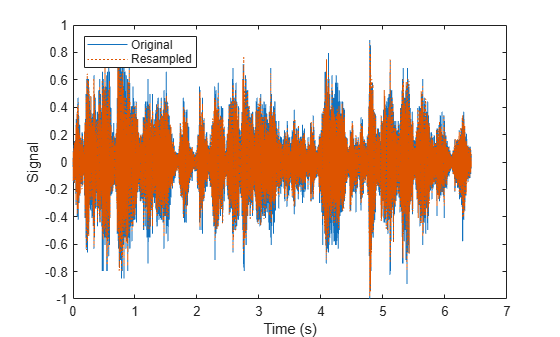# numpartitions

Return estimate for reasonable number of partitions for parallel processing

## Syntax

``n = numpartitions(sds)``
``n = numpartitions(sds,pool)``

## Description

example

````n = numpartitions(sds)` returns the default number of partitions for the signal datastore `sds`.```

example

````n = numpartitions(sds,pool)` returns a reasonable number of partitions to parallelize `sds` over the parallel pool. If `sds` contains file data, the number of partitions depends on the number of workers in the pool and the total number of files.If `sds` contains in-memory data, the number of partitions depends on the number of workers in the pool and the total number of members. To parallelize datastore access, you must have Parallel Computing Toolbox™ installed.```

## Examples

collapse all

Specify the file path to the example signals included with MATLAB®. Create a signal datastore that points to the specified folder.

```folder = fullfile(matlabroot,'toolbox','matlab','audiovideo'); sds = signalDatastore(folder,'SampleRateVariableName','Fs');```

Get the default number of partitions for the signal datastore.

`n = numpartitions(sds)`
```n = 7 ```

Partition the datastore into the default number of partitions and return the datastore corresponding to the fourth partition.

`subsds = partition(sds,n,4);`

Use the `extractAfter` function to display the name of the file contained in the datastore corresponding to the fourth partition.

`fName = extractAfter(subsds.Files,'audiovideo\')`
```fName = 1x1 cell array {0x0 char} ```

Read the data and information about the signal in the datastore corresponding to the fourth partition. Extract the sample rate from `info` and resample the signal to half the original sample rate. Plot the original and resampled signals.

```while hasdata(subsds) [data,info] = read(subsds); fs = info.SampleRate; f_res = 0.5*fs; ts = (0:length(data)-1)/fs; data_res = resample(data,1,2); t_res = (0:length(data_res)-1)/f_res; plot(ts,data,t_res,data_res,':') xlabel('Time (s)') ylabel('Signal') legend('Original','Resampled','Location','NorthWest') end```Specify the path to a directory containing example signals included with MATLAB®.

`folder = fullfile(matlabroot,'toolbox','matlab','audiovideo');`

Create a signal datastore that points to the specified folder.

`sds = signalDatastore(folder);`

Return an estimate for a reasonable number of partitions for parallel processing, given the current parallel pool.

`pool = gcp;`
```Starting parallel pool (parpool) using the 'local' profile ... Connected to the parallel pool (number of workers: 6). ```
`n = numpartitions(sds,pool)`
```n = 7 ```

Partition the signal datastore and read the signal data in each part.

```parfor ii = 1:n subds = partition(sds,n,ii); while hasdata(subds) data = read(subds); end end```

## Input Arguments

collapse all

Signal datastore, specified as a `signalDatastore` object.

Parallel pool, specified as a parallel pool object.

## Output Arguments

collapse all

Number of partitions over which datastore access is parallelized. By default, the number of partitions is min(Nobservations , 3Nworkers), where:

• Nobservations is the number of files in the datastore (in case of file data) or number of members in the datastore (in case of in-memory data).

• Nworkers is the number of workers in the pool.